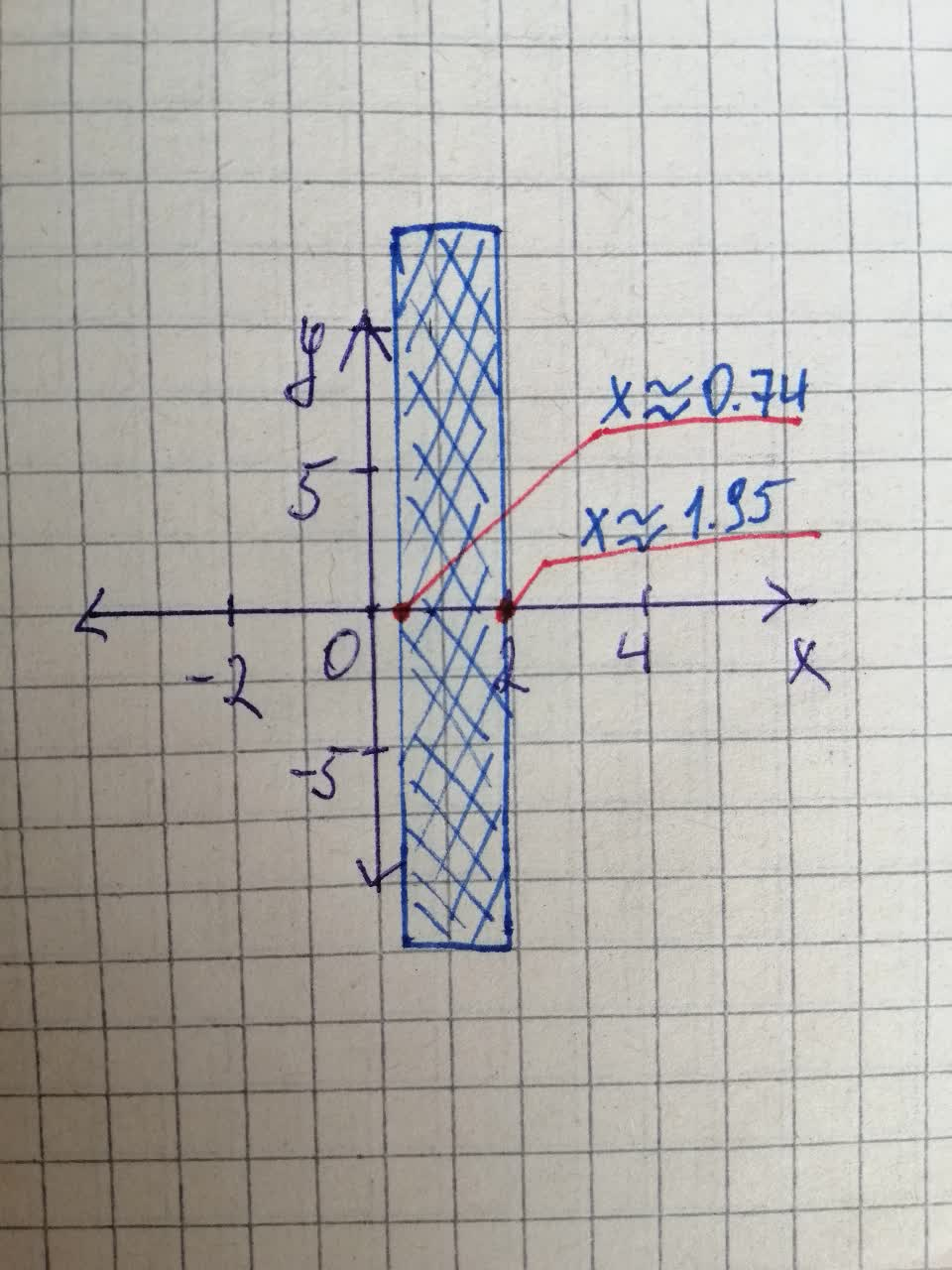Question# Solving Inequalities graphically: Use a graphing device to solve the inequality x^{4}+x^{3}\leq5x^{2}+4x-5

Decimals
ANSWEREDSolving Inequalities Graphically: Use a graphing device to solve the inequality. Express your answer using interval notation, with the endpoints of the interval rounded to two decimals.
$$\displaystyle{x}^{{{4}}}+{x}^{{{3}}}\leq{5}{x}^{{{2}}}+{4}{x}-{5}$$2021-08-11
Step 1
Consider the following inequality,
$$\displaystyle{x}^{{{4}}}+{x}^{{{3}}}\leq{5}{x}^{{{2}}}+{4}{x}-{5}$$
Subtract both sides of the equation $$\displaystyle{5}{x}^{{{2}}}+{4}{x}-{5}$$ to get the following,
$$\displaystyle{x}^{{{4}}}+{x}^{{{3}}}-{5}{x}^{{{2}}}-{4}{x}+{5}\leq{0}$$
By using software to get the value of $$\displaystyle{x}\approx{0.74},\ {x}\approx{1.95}$$
Use graphing device to get the following,From the above graph $$\displaystyle{x}\in{\left[{0.74},\ {1.95}\right]}$$
So, the solution is $$\displaystyle{x}\in{\left[{0.74},\ {1.95}\right]}$$